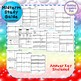Editable Algebra 1 Midterm, Final Exam (3 versions) and study guidesSubject
Resource Type
Product Rating
4.0
6 Ratings
File Type

Compressed Zip File

7 MB
Share
Product Description

This item is included in the following bundles I offer:

This item includes the following:

1. Midterm Study Guide (97 Questions)

2. Midterm (50 Questions)

3. Final Exam Study Guide (170 Questions)

4. Final Exam (100 Questions)

The final is available in 3 versions. There is one multiple choice, one short answer, and one mixed version. Note the questions are the same but the format is different among the 3 versions.

Are these editable?? Yes! The midterm, study guides, and 2 versions of the final are editable in PowerPoint and Google Slides. The mixed version is only provided in PDF form, however, you can delete answer choices for any questions in the multiple choice version and create another if you'd like.

The midterm includes the following topics:

Algebra Expressions & The Real Number System Lessons Notes Homework Quiz & Test

Unit 1 Lessons:

Lesson 1 Simplifying & Evaluating Expressions

Lesson 2 Translating Verbal Phrases into Expressions

Lesson 3 The Real Number System

Lesson 4 Adding & Subtracting Real Numbers

Lesson 5 Multiplying Real Numbers

Lesson 6 Dividing Real Numbers

Study Guides & Editable Quiz and Test

Algebra Equations & Inequalities Lesson Notes Homework Quiz & Test

Unit 2 Lessons:

Lesson 1 One and Two Step Equations

Lesson 2 Multi-Step Equations

Lesson 3 One and Two Step Inequalities

Lesson 4 Multi-Step Inequalities

Lesson 5 Solving Proportions

Lesson 6 Word Problems and Applications

Lesson 7 Absolute Value Equations

Lesson 8 Absolute Value Inequalities

Algebra Relations & Functions Lesson Notes Homework Quiz & Test

Unit 3 Lessons:

Lesson 1 Relations vs. Functions

Lesson 2 Function Tables & Rules

Lesson 3 Graphing Functions

Lesson 4 Evaluating Functions

Lesson 5 Zeros of Functions

Algebra Slope & Writing Equations of Lines Lesson Notes Homework Quiz & Test

Unit 4 Lessons:

Lesson 1 Slope

Lesson 2 Writing Linear Equations in Slope-Intercept Form

Lesson 3 Standard form to Slope Intercept Form

Lesson 4 Writing Linear Equations in Slope-Intercept Form

given a point and a slope or given two points

Lesson 5 Parallel & Perpendicular Lines

Lesson 6 Graphing Linear Equations

Lesson 7 Word Problems & Applications

Lesson 8 Scatter Plots & Lines of Best Fit

Algebra Systems of Equations Lesson Notes Practice Homework & Quiz

Unit 5 Lessons:

Lesson 1: Solving Systems of Equations by Graphing

Lesson 2: Solving Systems of Equations by Substitution

Lesson 3: Solving Systems of Equations by Elimination

Lesson 4: Solving Systems of Equations by Applications

Lesson 5: Solving Systems of Equations by Special Cases

Study Guides & Editable Quiz and Test

Exponent Rules with Monomials Lesson Notes BUNDLE Homework Quiz & Test

Unit 6 Lessons:

Lesson 1 Monomials

Lesson 2 Multiplying Monomials

Lesson 3 Dividing Monomials

Lesson 4 Powers of Monomials

Lesson 5 Negative Exponents

Lesson 6 More with Negative Exponents

Lesson 7 Scientific Notation

The final includes all of the above and also:

Algebra Polynomials BUNDLE Lesson Notes Practice Homework Quiz & Test

Unit 7 Lessons:

Lesson 1 Classifying Polynomials and Addition/Subtraction of Polynomials

Lesson 2 Multiplying Polynomials

Lesson 3 Dividing Polynomials

Lesson 4 Multi-Step and Applications with Polyn0mials

Algebra Factoring Polynomials BUNDLE Lesson Notes Practice Homework Quiz & Test

Unit 8 Lessons:

Lesson 1 Greatest Common Factor

Lesson 2 x2 + bx + c

Lesson 3 ax2 + bx + c

Lesson 4 Difference of two perfect squares and perfect square trinomials

Lesson 5 Grouping

Lesson 6 Factoring Completely

Algebra Quadratic Equations BUNDLE Lesson Notes Practice Homework Quiz & Test

Unit 9 Lessons:

Lesson 1 Introduction to Quadratic Equations

Lesson 3 Quadratic Equations in Vertex Form

Lesson 4 Solving Quadratic Equations by Graphing

Lesson 5 Solving Quadratic Equations by Factoring

Lesson 6 Solving Quadratic Equations by Finding Square Roots

Algebra Rational Expressions & Equation Lesson Notes Homework Quiz & Test

Unit 10 Lessons:

Lesson 1 Simplifying Rational Expressions

Lesson 2 Multiplying Rational Expressions

Lesson 3 Dividing Rational Expressions

Lesson 4 Adding & Subtracting Rational Expressions with Like Bases

Lesson 5 Adding & Subtracting Rational Expressions with Unlike Bases

Lesson 6 Applications

Lesson 7 Solving Rational Equations

Lesson 8 Solving Rational Equations with Extraneous Solutions

Algebra Radical Expressions & Equation Lesson Notes Homework Quiz & Test

Unit 11 Lessons:

Lesson 2 Cube Roots

Lesson 3 Simplifying Square Roots with Variables

Lesson 4 Simplifying Cube Roots with Variables

Lesson 7 Dividing Radicals Part 1

Lesson 8 Dividing Radicals Part 2

Lesson 10 Applications

Algebra Probability & Statistics 10 Lessons Notes Homework Quiz & Test BUNDLE

Unit 12 Lessons:

Lesson 1 Simple Probability

Lesson 2 Theoretical vs. Experimental Probability

Lesson 3 Permutations

Lesson 4 Combinations

Lesson 5 Probability using Permutations & Combinations

Lesson 6 Compound Events

Lesson 7 Surveys & Samples

Lesson 8 Measures of Central Tendency

Lesson 9 Stem & Leaf Plots, Histograms & Box & Whisker Plots

Lesson 10 Scatter Plots & Lines of Best Fit

Interested in my curriculum containing all of these units?

Algebra 1 curriculum BUNDLE Lesson Notes Homework Study Guides Quizzes & Tests

Prefer the curriculum with GROWING GAME PACK?

Algebra 1 curriculum BUNDLE with growing GAME PACK

Check out Making Algebra Fun for individual games.

The purchase of this product entitles you to personal use within one classroom. Sharing among colleagues is not permitted. Posting online or selling is strictly prohibited. Thank you for respecting my time and work.

Total Pages
N/A